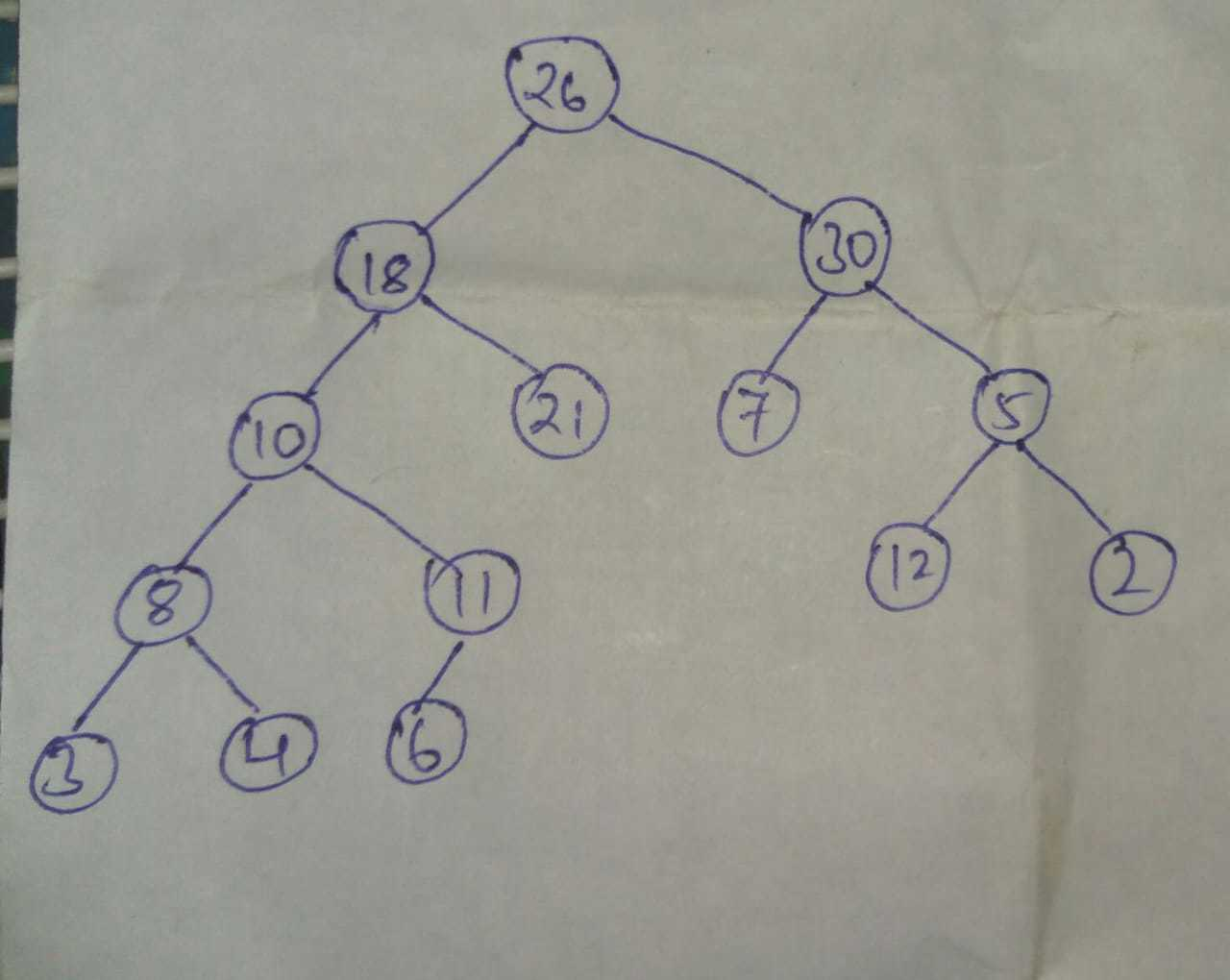# Josh Interview Experience | Pool Drive

• Difficulty Level : Hard
• Last Updated : 02 Aug, 2021

After Clearing Written Round <a href=”https://www.geeksforgeeks.org/josh-technology-group-recruitment-process-pool-drive/”>JOSH TECHNOLOGY RECRUITMENT PROCESS</a>, I was Called for the Interviews.

For every round, interviewer asked the logic for each question, then he told me to code it on paper and dry run the code for some inputs.

Technical Interview Round 1: (1.30 hr)

Q.1) Given a binary tree, print the node values in any order which follow SpecialSum Property.

SpecialSum Property is having sum of all the left childs only, equal to the sum of all the right childs only.

Note– all leaf nodes are not following SpecialSum Property.

I/P-O/P – 10, 18, 30

Explanation–

Node 10 has left sum=8+3(only left childs)=11 and right sum=11(only right childs)

Node 18 has

t sum=10+8+3=21 and right sum=21

Node 30 has left sum=7 and right sum=5+2=7

Q.2) Given a valid expression in linked list, print the number of redundant parentheses.

Note– Dont reduce (a+b) to a+b

I/P – ((a-b)+(((c+d)))+(e*f))

O/P – 2

Explanation– (((c+d))) can be reduced to (c+d)

Technical Interview Round 2:(1.30 hr)

Q.1) Given a binary tree, Print the right view of the tree.

/”>Right View</a>

Q.2) Given a array of size N and a element K, we have to rearrange the element in such a way that all the element values less than K are at beginning (in any order) followed by all the elements values greater than K (in any order) and all the element having value equal to K are at the end.

I/P- [6, 7, 2, 5, 4, 9, 8, 5], k=5

O/P- [2, 4, 6, 7, 9, 8, 5, 5]

// i answered the solution in O(n) time complexity, O(1) space and 2 traversal, then he told me to do it in 1 traversal. I answered after thinking 2-3 min.

Q.3) Given a binary search tree and a range [L, R], delete all elements which are not in the range.

Link– <a href=”https://www.geeksforgeeks.org/remove-bst-keys-outside-the-given-range/”>Remove outside range elements From BST</a>

Technical Interview Round 3: (2 hr)

Q.1) Given a head node of binary tree and a node K, print the leng

f closest leaf node from node K.

I told the answer in 2 traversal, then he replied to solve in 1 traversal. he also helped me to write the final optimised dcode

Q.2) Given a array of size N, how much water can be stored in between them.

I told the solution in O(N) time, O(2N) Space and 2 traversal, then the interviewer asked me to optimise the space

I answered with O(N) time, O(N) Space and 2 traversal,  then the interviewer asked me to solve it without the space.

I answered with O(N) time, O(1) Space and 2 traversal,  then the interviewer told the hint for 1 traversal.

Personal Interview Round 1: (10-15 mins)

Interviewer asked me some quick questions like-

• Introduction.
• Environment of geeksforgeeks training classes that i mentioned in my resume.
• about platforms which i used to code.
• i have mentioned c and c++ languages, he asked me why i haven’t learned java or any other languages.
• projects which i mentioned.
• about team projects which i worked.
• Current semester subjects.
• some questions with DBMS and OS.
• last round final question and solution.
• hobbies and interest.

finally asked any question that i have for them.

HR Round : (5 mins)

• Introduction.
• Hows the day and why it is so.
• how much i like competitive coding and how i got the interest in it.
• about other companies that i was having offers.
• finally asked any question that i have for them.
• then congrats me and told me that i am selected.

// Every Round interviewer was very friendly. Very good experience.

// By Pratik Agarwal

My Personal Notes arrow_drop_up